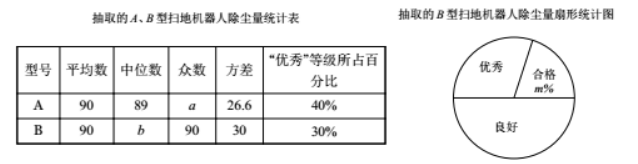10 台 $A$ 型挸地机呮人的除尘量: $83,84,84,88,89,89,95,95,95,98$.
10 台 $B$ 型扫地机器人中“良好”等级包含的所有数据为: $85,90,90,90,94$(1) 填空: $a=\_\_, b=\_\_, m=\_\_$
(2)这个月公可生产 $B$ 型扫地机器人共 3000 台, 估计该月 $B$ 型扫地机器人“优秀”等级的台数；
(3)根捃以上数据,你认为该公司生产的嗎种型号的扫地机器人扫地质量更好? 诮说明理由（写出一条 理由即可).

(1)$95,90,20$
(2) $3000 \times 30 \%=900$ 台
(3) $A$ 型号更好, 在平均数均为 90 的情况下, $A$ 型号的平均除坐量㧿数 $95 > B$ 型号的平均除坐量众数 90
①点击 首页查看更多试卷和试题 , 点击查看 本题所在试卷